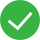Documentation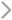GPU Elastic ComputeUser GuideInstallation guideInstall TensorFlow

# Install TensorFlow

Last updated：2020-12-10 11:21:34

### Install TensorFlow

#### Online installation

Verify that the network connection is operating properly and execute the following command:

``````pip3 install --upgrade tensorflow-gpu
``````

#### Install TensorFlow by using the source code

1. Access TensorFlow 1.5.0 or copy the https://github.com/tensorflow/tensorflow link to the web browser address bar.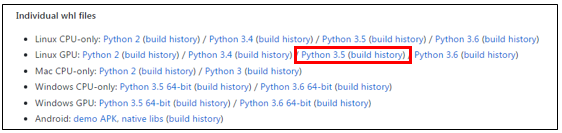3. Execute the following command to install TensorFlow:

``````pip3 install tf_nightly_gpu-1.head-cp35-cp35m-linux_x86_64.whl
``````

### Verify the installation

After you install TensorFlow, execute a Python script file that contains the following code to verify the installation:

``````import tensorflow as tf
import numpy as np
x_data = np.float32(np.random.rand(2, 100))
y_data = np.dot([0.100, 0.200], x_data) + 0.300
b = tf.Variable(tf.zeros())
W = tf.Variable(tf.random_uniform([1, 2], -1.0, 1.0))
y = tf.matmul(W, x_data) + b
loss = tf.reduce_mean(tf.square(y - y_data))
train = optimizer.minimize(loss)
init = tf.initialize_all_variables()
sess = tf.Session()
sess.run(init)
for step in range(0, 201):
sess.run(train)
if step % 20 == 0:
print (step, sess.run(W), sess.run(b))*
``````

If TensorFlow has been installed successfully, the following information is displayed: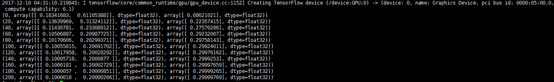Did you find the above information helpful?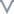UnhelpfulMostly UnhelpfulA little helpfulHelpfulVery helpful

#### What might be the problems?

Insufficient
Outdated
Unclear or awkward
Redundant or clumsy
Lack of context for the complex system or functionality

#### More suggestions

0/200